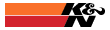Home Exhaust/Manifolds Intake Items Turbo Items Chassis/Suspension Custom Fabs RD Merchandise Spare Parts Custom PaymentsRD Fabs Tech Center Cart Total: \$

# Tech Calculators

 Calculate HP from Trap Speed Vehicle weight in pounds (including driver)lbs Trap speed at end of 1/4 mi in m.p.h.m.p.h. You are making : HP at the rear wheels and HP at the flywheel*.
*Note:  Assuming a 18% drive train loss.

 Calculate HP from ET Vehicle weight in pounds (including driver)lbs ET in secondssec You are making : HP at the rear wheels and HP at the flywheel*.

*Note:  Assuming a 18% drive train loss.

 Calculate Tire Diameter Tire width - Series - Wheel DiameterExample 275 - 60 R 15 Tire Width Tire Series Wheel Diameter Tire diameter in inches

 Calculate mph for RPM range Tire diameter Gear Ratio Forced PSI assumes the car's transmission is in drive or 1:1 ratio, no overdrive. For Overdrive cars mutliply the overdrive ratio times the rear and gear ratio. Example a 700R4 transmission has an overdive ratio 0.7:1. For a 4.11 rear end multiply 4.11 x 0.7 = 2.877. Enter 2.877 as the number for the gear ratio to calculate speeds in overdive. 1000 rpm mph 4500 rpm mph 1500 rpm mph 5000 rpm mph 2000 rpm mph 5500 rpm mph 2500 rpm mph 6000 rpm mph 3000 rpm mph 6500 rpm mph 3500 rpm mph 7000 rpm mph 4000 rpm mph 7500 rpm mph

 Calculate Rear End Gear Ratio Enter the tire diameter in inches, rpm's of the motor at a certain speed as indicated by your speedometer in mph. Forced PSI assumes the car's transmission final drive ratio is 1:1, no overdrive. Tire Diameter (in) RPM Speed at RPM (mph) Rear End Gear Ratio